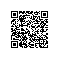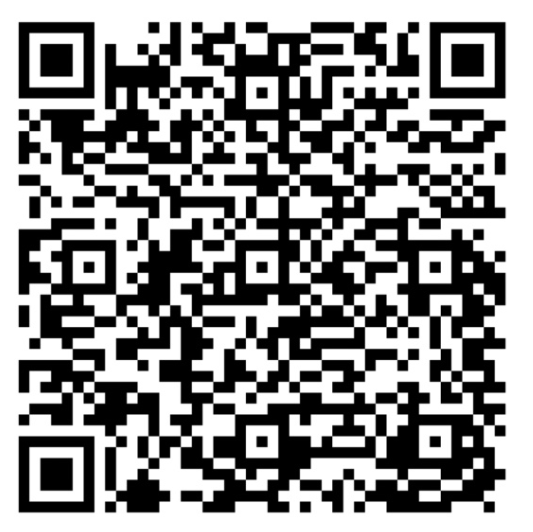# POJ 2260（ZOJ 1949） Error Correction 一个水题

Description

A boolean matrix has the parity property when each row and each column has an even sum, i.e. contains an even number of bits which are set. Here’s a 4 x 4 matrix which has the parity property:
1 0 1 0

0 0 0 0

1 1 1 1

0 1 0 1

The sums of the rows are 2, 0, 4 and 2. The sums of the columns are 2, 2, 2 and 2.
Your job is to write a program that reads in a matrix and checks if it has the parity property. If not, your program should check if the parity property can be established by changing only one bit. If this is not possible either, the matrix should be classified as corrupt.

Input

The input will contain one or more test cases. The first line of each test case contains one integer n (n<100), representing the size of the matrix. On the next n lines, there will be n integers per line. No other integers than 0 and 1 will occur in the matrix. Input will be terminated by a value of 0 for n.
Output

For each matrix in the input file, print one line. If the matrix already has the parity property, print “OK”. If the parity property can be established by changing one bit, print “Change bit (i,j)” where i is the row and j the column of the bit to be changed. Otherwise, print “Corrupt”.
Sample Input

4
1 0 1 0
0 0 0 0
1 1 1 1
0 1 0 1
4
1 0 1 0
0 0 1 0
1 1 1 1
0 1 0 1
4
1 0 1 0
0 1 1 0
1 1 1 1
0 1 0 1
0
Sample Output

OK
Change bit (2,3)
Corrupt

#include <iostream>
#include <stdio.h>
#include <string.h>
#include <stdlib.h>
using namespace std;

int main(){
int n,m;
bool s;
short int a,b;
bool a1,b1;
while(cin>>n&&n!=0){
int hang=0;
int lie=0;
for(int i=0;i<n;i++){
a[i]=0;
for(int j=0;j<n;j++){
scanf("%d",&s[i][j]);
a[i]+=s[i][j];
}
}
for(int j=0;j<n;j++){
b[j]=0;
for(int i=0;i<n;i++){
b[j]+=s[i][j];
}
}
for(int i=0;i<n;i++){
a1[i]=1;
b1[i]=1;
if(a[i]%2!=0){
a1[i]=0;
}
if(b[i]%2!=0){
b1[i]=0;
}
}
int flag1 = 0, flag2=0;
int hang1,lie1;
for(int i=0;i<n;i++){
if(a1[i]==0){
hang++;
if(flag1==0){
hang1=i+1;
}
}
if(b1[i]==0){
lie++;
if(flag2==0){
lie1=i+1;
}
}
}
if(hang==1&&lie==1){
printf("Change bit (%d,%d)\n",hang1,lie1);
}else if(hang==0&&lie==0){
printf("OK\n");
}
else{
printf("Corrupt\n");
}
}
return 0;
}使用钉钉扫一扫加入圈子
+ 订阅